# BEE Basic Electricity Electronics question paper December 2013

BEE Basic Electricity Electronics question paper December 2013 – The B.E.E question paper for Mumbai University students of semester 1.

### BEE Basic Electricity Electronics question paper December 2013

1 (a) Using source transformation Find I.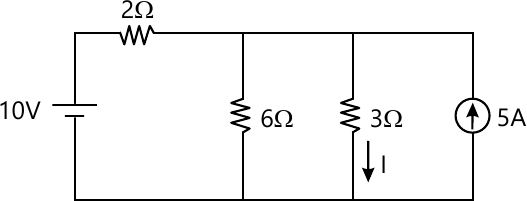3 M
1 (b) State and Explain Norton’s theorem.
3 M
1 (c) Derive an expression for the average value of a sinusoidally varying current in terms of Peak Value.
3 M
1 (d) Derive the condition for resonance in a series circuit.
3 M
1 (e) Give relation between line current and phase current, line voltage and phase voltage in balanced star and delta connected load.
2 M
1 (f) What are assumptions for an ideal transformer?
4 M
1 (g) Draw and explain circuit diagram for half wave rectifier.
2 M
2 (a) Find the currents I1, I2, I3 in the given circuit by node voltage method.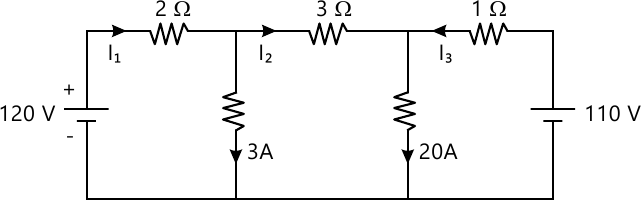6 M
2 (b) For the circuit shown determine the
1) Supply frequency (f)
2) Coil resistance (r)
3) Supply Voltage (v)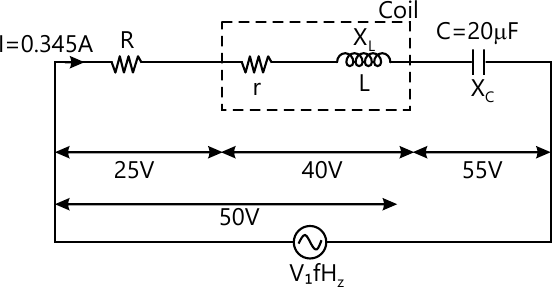8 M
2 (c) Draw and explain Phasor of I-Phase practical transformer when
6 M
3 (a) Find the value of circuit elements and reactive voltampere drawn for a balanced 3 phase load connected in delta and draws a power 12k W at 440V. The power factor is 0.7 leading.
8 M
3 (b) The following results were obtained on a 40 KVA, 2400/120 V transformer.
O.C. Test : 120V, 9.65A and 396W (on L.V.side)
S.C Test: 92V, 20.8A and 810 W (on H.V. side)
Calculate the parameter of approximate equivalent circuit referred to H.V side.
6 M
3 (c) Explain series inductor filter.
2 M
3 (d) Explian circuit diagram and working of CE configuration of BJT.
4 M
4 (a) Determine current through 20? resistor in the following circuit.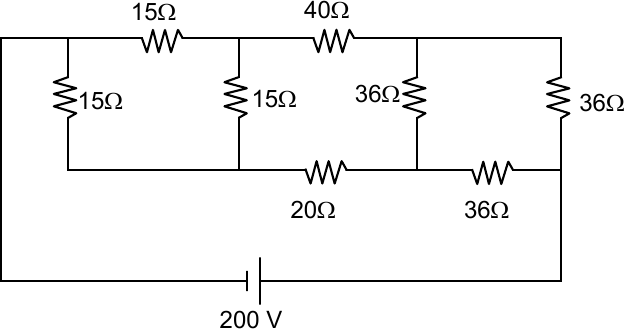7 M
4 (b) Two current are represented byI1=15Sin(wi+?3) and I2=25Sin (wt+?4).
these current are fed into common conductor. Find the total current. If the conductor has resistance 50? what will be energy loss in 10 hours.
5 M
4 (c) In a three phase power measurement by two waltmeter method , both the wattmeters read the same value. What is the power factor of the load? Justify your answer.
4 M
4 (d) Explain the circuit diagram and waveforms of Bridge rectifier
4 M
5 (a) For the given circuit find the value of RL so the maximum power dissipated in it. Also find Pmax.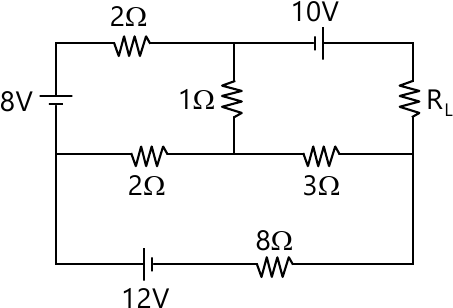8 M
5 (b) With proper phase diagrams, explain behaviour of a pure capacitor ina AC circuit.
4 M
5 (c) Derive condition for maximum efficiency of a trransformer. Also deive equation for load at maximum efficiency.
8 M
6 (a) Determine current in 1? resistor using superposition theorem.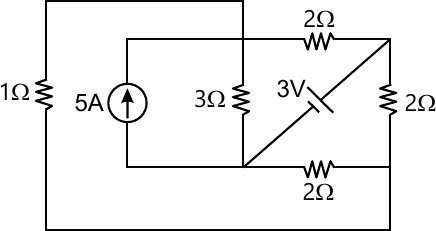7 M
6 (b) An inductive coil of resistance 10? and inductance. 0.1 H is connected in parallel with 150 ?F capacitor to a variable frequency, 200V supply. Find the resonance frequency at which the total current taken from supply is in Phase with supply voltage. Also find value of this current. Draw the phasor diagram.
7 M
6 (c) Two wattmeters are connected to measure power in a phase circuit. The reading of one of the wattmeter is 7 kW when load power factor is unity. If the power factor of the load is changed to 0.707 lagging without changing the total input power, calculate the readings of the two wattmeters.
6 M